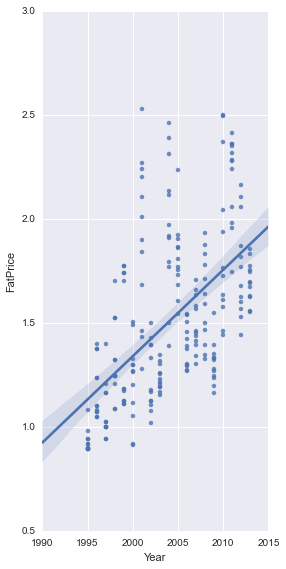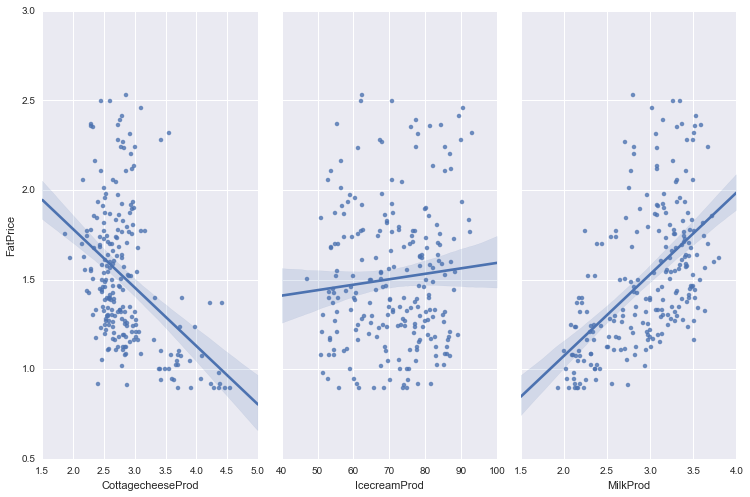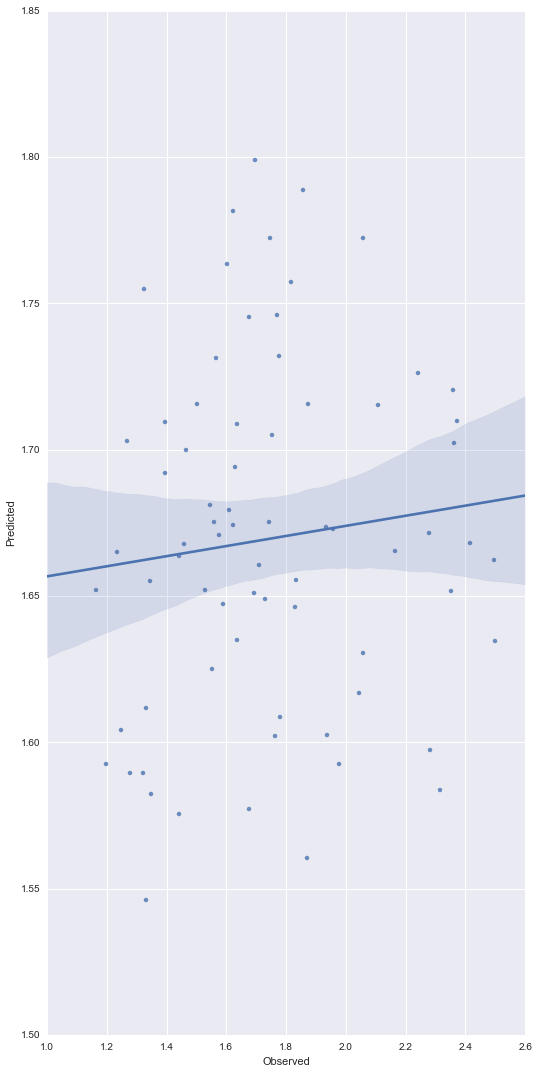DairyData

### Regression Model¶

In this post, I will built a linear regression model to predict the fat price using the production of cottage cheese, icecream, and milk. The data can be dowloaded from https://courses.edx.org/courses/course-v1:Microsoft+DAT203x+1T2016/info.

In :
# import python packages
import pandas as pd
import seaborn as sns
import statsmodels.formula.api as sm
from sklearn.linear_model import LinearRegression
from sklearn import metrics
from sklearn.cross_validation import train_test_split
import numpy as np
import matplotlib.pyplot as plt

# allow plots to appear directly in the notebook
%matplotlib inline

In :
dairy = pd.read_csv("cadairydata.csv", index_col=0)

In :
dairy = dairy[["Year", "Month", "CottagecheeseProd", "IcecreamProd", "MilkProd", "FatPrice"]]

In :
dairy.head(3)

Out:
Year Month CottagecheeseProd IcecreamProd MilkProd FatPrice
1 1995 Jan 4.370 51.595 2.112 0.9803
2 1995 Feb 3.695 56.086 1.932 0.8924
3 1995 Mar 4.538 68.453 2.162 0.8924
In :
sns.pairplot(dairy, x_vars="Year", y_vars="FatPrice", size=8, aspect=0.5, kind='reg')

Out:
<seaborn.axisgrid.PairGrid at 0x1f9ead30>Generally speaking, fat prices seems to increase over the years. However, the variance is huge.

In :
# visualize the relationship between the features and the response
sns.pairplot(dairy, x_vars=["CottagecheeseProd", "IcecreamProd", "MilkProd"], y_vars="FatPrice", size=7, aspect=0.5, kind='reg')

Out:
<seaborn.axisgrid.PairGrid at 0x1fcd8b70>It seems that the fat price decreases with increase in cottage cheese production and increases with milk production.

Now, let's build a multivariate linear regression model to predict fat price.

In :
dairy.shape

Out:
(228, 6)
In :
# divide the data into training and testing datasets
np.unique(dairy['Year'])

Out:
array([1995, 1996, 1997, 1998, 1999, 2000, 2001, 2002, 2003, 2004, 2005,
2006, 2007, 2008, 2009, 2010, 2011, 2012, 2013], dtype=int64)
In :
train = dairy[dairy['Year'] < 2008]
test = dairy[dairy['Year']>=2008]
train.shape, test.shape

Out:
((156, 6), (72, 6))
In :
## Apply SCIKIT LEARN

# create x and y
features_used = ["CottagecheeseProd", "IcecreamProd", "MilkProd"]
x = train[features_used]
y = train.FatPrice

# fit a regression model
lm_model = LinearRegression()
lm_model.fit(x, y)

# print summary of the model

Out:
LinearRegression(copy_X=True, fit_intercept=True, n_jobs=1, normalize=False)
In :
# coefficients of the model
print'Coefficients:'
print ('Intercept: ' + str(lm_model.intercept_))
zip(features_used, lm_model.coef_)

Coefficients:
Intercept: 0.802389807794

Out:
[('CottagecheeseProd', -0.090395152609967278),
('IcecreamProd', -0.0016630176241065734),
('MilkProd', 0.35822753455502709)]
In :
#coefficient of detemination of the training dataset
print 'R-squared: %0.2f'% lm_model.score(x,y)

R-squared: 0.25


Looking at the coefficients, MilkProd is the most significant factor. A unit milk production would increase fat price by a factor of 0.35. The other two features are less significant. Looking at the coeficient of determination, R-squared value is 0.25 doesn't show a strong model.

Let's build a regression model with only MilkProd as independent feature.

In :
features_used1 = [ "MilkProd"]
x1 = train[features_used]
y1 = train.FatPrice

In :
# fit a regression model
lm_model1 = LinearRegression()
lm_model1.fit(x1, y1)

Out:
LinearRegression(copy_X=True, fit_intercept=True, n_jobs=1, normalize=False)
In :
# coefficients of the model
print'Coefficients:'
print ('Intercept: ' + str(lm_model1.intercept_))
zip(features_used1, lm_model1.coef_)

Coefficients:
Intercept: 0.802389807794

Out:
[('MilkProd', -0.090395152609967278)]
In :
#coefficient of detemination of the training dataset
print 'R-squared: %0.2f'% lm_model1.score(x1,y1)

R-squared: 0.25


There is difference is the R-squared value.

In :
#plt.plot(train, lm_model.predict(train), color='blue',linewidth=3)


### Model Evaluation¶

Mean Absolute Error (MAE) is the mean of the absolute value of the errors:

$$\frac 1n\sum_{i=1}^n|y_i-\hat{y}_i|$$

Mean Squared Error (MSE) is the mean of the squared errors:

$$\frac 1n\sum_{i=1}^n(y_i-\hat{y}_i)^2$$

Root Mean Squared Error (RMSE) is the square root of the mean of the squared errors:

$$\sqrt{\frac 1n\sum_{i=1}^n(y_i-\hat{y}_i)^2}$$
In :
testing = test[features_used]
testing_y = test.FatPrice
test_predicted= lm_model.predict(testing)
Predicted_result = pd.DataFrame({"Observed":testing_y,"Predicted":test_predicted})
Predicted_result.head()

Out:
Observed Predicted
157 1.4645 1.699825
158 1.3452 1.655022
159 1.3252 1.755014
160 1.3948 1.692029
161 1.4999 1.715517
In :
# calculate MAE using scikit-learn
print metrics.mean_absolute_error(testing_y, test_predicted)

0.277952373732

In :
# coffient of determination for the testing dataset
print 'R-squared: %0.2f'% lm_model.score(testing, testing_y)

R-squared: -0.04

In :
# calculate MSE using scikit-learn
print metrics.mean_squared_error(testing_y, test_predicted)

0.125656951137

In :
# calculate RMSE using scikit-learn
print np.sqrt(metrics.mean_squared_error(testing_y, test_predicted))

0.354481242293


Plot of the fitted model for the testing dataset

In :
sns.pairplot(Predicted_result, x_vars='Observed', y_vars='Predicted', size=15, aspect=0.5, kind='reg')

Out:
<seaborn.axisgrid.PairGrid at 0x203602b0>In [ ]:
# the same plot can be created using
#plt.scatter(Predicted_result.Observed, Predicted_result.Predicted,  color='blue')
#plt.xticks(())
#plt.yticks(())
#plt.show()


This is not an ideal fit. This is one of those examples in which linear regression is not a good model to predict with. In the next section, I will apply support vector machine(svm) to see if I can get a better model.